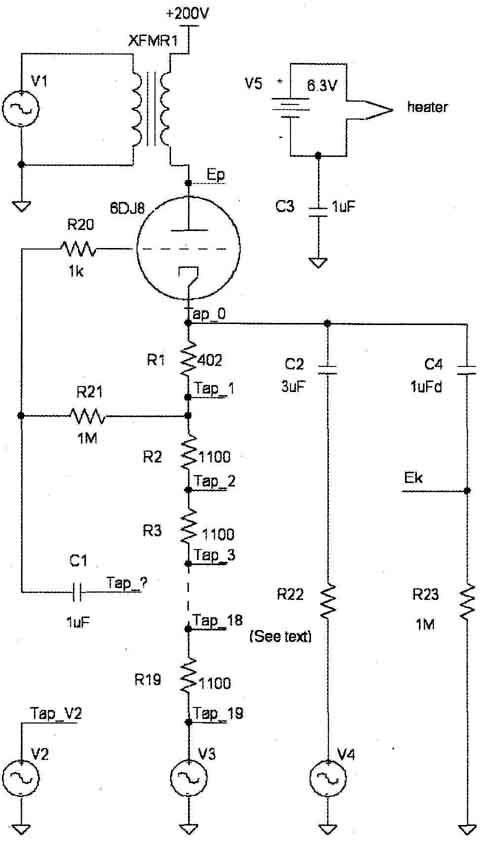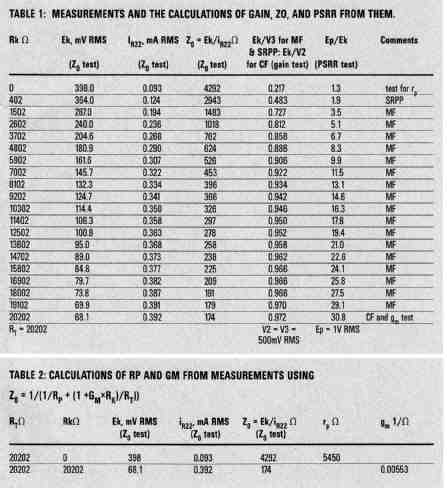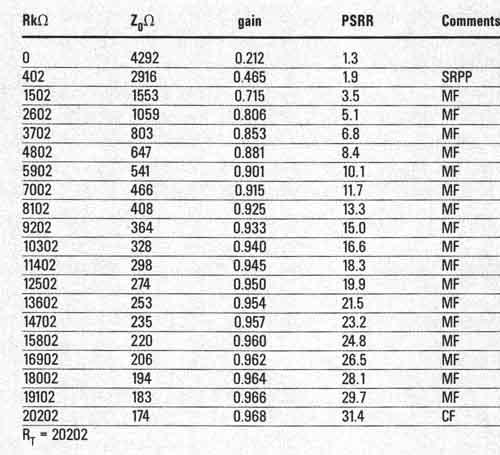# The Cathode Follower and Its Weaker Siblings

Test results confirm that cathode followers are even more capable of driving capacitive loads than equivalent common-cathode amplifiers.

In a recent article (“Rehabilitating Cathode Followers”), I compared the abilities of the Cathode Follower (CF) and the Common Cathode Amplifier (CCA) to drive capacitive loads. I showed that CFs and CCAs made from identical triodes biased to identical plate voltages and currents and driving identical loads “disconnected” from their loads at the same peak currents and voltages—one on the load voltage downswing, and the other on the upswing. The article also discussed that the higher output impedance of the CCA left it at a disadvantage when it came to driving capacitive loads—that is, attenuation occurred more readily at higher frequencies for the CCA.

In a subsequent exchange of private letters, it was pointed out that the CCA could address this deficit by paralleling resistances across a capacitive load (thus extending bandwidth) and then increasing drive to reestablish the original level. However, it also became clear that these higher drive levels would increase distortion at the mid and low frequencies and limit the peak voltage across the load. It seemed that the CF still retained the advantage.

If the CF is the preferred driver for capacitive loads, it makes sense to more fully explore and understand its characteristics, which you should consider in comparison to those of CF-like circuits that nevertheless operate distinctly differently from a CF. Over the years, I have noticed that some people attribute CF-like characteristics to many CF-like circuits, apparently mainly because their outputs are cathodes. Recently, I found a manufacturer selling a preamp with an SRPP output stage that claimed to have a CF-like output impedance. The manufacturer subsequently confirmed by measurement, to his surprise, that the SRPP does not have the output impedance of a CF, and changed the specification accordingly. A similar assertion regarding an SRPP with a “low output impedance” was made even more recently. I’d like to explore this and other differences between the CF and its variants in the rest of this article.

THE CIRCUITS UNDER INVESTIGATION

There’s value both in performing circuit derivations and in building and measuring the circuit on the bench. There’s no denying bench results, but a validated derivation is priceless when it comes to getting a feel for how a circuit works and for applying and optimizing it. So I’ll start with a general-purpose schematic (Fig. 1) that illustrates the CF and its variants and how to test them.

I’ll present measurements and calculations I made from them in Tables 1 and 2 to determine certain circuit parameters of interest. I’ll also provide calculations (Table 3) of the same parameters using the equations I derived in the sidebar. If you’re not interested in the derivations, you can skip the sidebar, although I think you’ll gain some insight if you review it. I’ll compare and discuss the results of the measurements and the de rived equations later.FIGURE 1: Circuit for testing a CF, and SRPP or an MF.

In Fig. 1, 1100-ohm resistors R2-R19 are strung together with 402-ohm R1. You can conveniently connect each point between a connected pair of resistors to Tap_? (one side of C1), affording a means to test different cathode follower (CF) variants. A connection of Tap_? to Tap_V2 produces a cathode follower. A connection to Tap_1 yields the top of an SRPP (although in this case, C1 is not really needed). Connections to Taps 2- 19 yield the top of a Mu Follower (MF), while a connection to Tap_O provides a convenient means of measuring the r_p (plate resistance) of the 6DJ8, as I’ll show.

For the SRPP and MF, think of V3 and the resistors between it and the connection to Tap_? as the controlled source and the resistance seen at the plate of the bottom triode in those two-triode configurations. With the CF, V3 is uniformly 0 (V2 is the active source), and the resistors from R1-R19 are simply cathode resistors. Note that the DC bias of the triode is fixed for all variants at a 5mA plate current and a 100V plate voltage with the 6DJ8 I used.

Only one of the very low impedance (under 10-ohm) sources V1-V4 supplies a signal at any time; consider the remainder to be short circuits. These 1kHz signal sources are used to support the measurement of three important parameters of the circuits I’m examining.

GAINS OUTPUT IMPEDANCE, AND POWER-SUPPLY REJECTION RATIO (PSRR)

These three parameters represent excel lent figures of merit for a circuit. Consider the equations (derived in the side-bar) which determine them in the Fig. 1 circuit. Capacitors are assumed to be AC shorts and the 1M resistors are AC open circuits. RT is defined as the sum of R1 + R2 +. . . R19. For SRPPs and MFs, Rk is the value of that portion of RT between Tap_U and the selected connection (Tap_0, Tap_1,. . . Tap_19) to Tap_?. For CFs, Rk = R rp and g, are the plate resistance and transconductance, respectively, of the 6DJ8.

Measure output impedance (Z by first activating V4 and setting R22 to a finite, non-zero value (I used 1100-ohm in my measurements, but its value is unimportant in the derivation). Other sources are off and E is shorted to +200V. Determine Z by the ratio of the cathode voltage to the cathode current fed through R22 and C2, as was derived in the sidebar:

Z (measured) = Ek/I_R22

Z (derived) = 1/(1/r_p + (1 +g_m x Rk)/R_T)

TABLE 1: MEASUREMENTS AND THE CALCULATIONS OF GAIN, ZO, AND PSRR FROM THEM.

TABLE 2: CALCULATIONS OF HP AND GM FROM MEASUREMENTS USING = 1/(1/R + (1 GMxRK)/RT)}The Power-Supply Rejection Ratio (PSRR) specifies how well power-sup ply noise is rejected at the circuit output. Measure it by first activating V1. Other sources are off and R22 is removed.

PSRR is determined by the ratio of E Ek (which is always greater than 1 and improves as it increases in value). I also derived PSRR in the sidebar:

PSRR (measured) = EP/Ek

PSRR (derived) = r_p/Z_0

Measure gain by first activating source V2 for a CF or V3 for an SRPP or an MF. Other sources are set to 0, Ep is shorted to +200V, and R22 is removed.

Gain is determined by the ratio of Ek to the level of the active source (V2 or V3) and was derived in the sidebar:

GainMS (measured) = Ek/V2

GainMS (derived) = ((1 + g Rk)/RT) x Z_0

… for the MF and SRPP and ….

GainCF (measured) = Ek/V3

GainCF (derived) = g_m x Z_0

for the CF.

TABLE 3: CALCULATIONS OF GAIN, ZO, AND PSRR FROM THE SIDEBAR DERIVATIONS, USING TABLE 2 VALUES OF R AND GM.FIGURE 2: Measured and derived values of output impedance vs. Rk/RT.FIGURE 3: Measured and derived values of gain vs. Rk/RT.FIGURE 4: Measured and derived values of PSRR vs. Rk/Rr

MEASUREMENTS AND CALCULATIONS

A summary of the measurements I made is given in the Table (above), which also contains the values of the parameters Gain, Zo, and PSRR calculated from those measurements. You can also calculate those same parameters (Table 3) from their derivations if you first calculate g and r (Table 2) from certain measurements listed in Table 1. (You could read g and rp from a chart of plate curves, but it is better to use the values of the tube under test to properly compare measured and derived results.) Comparing calculations from the measurements and the derivations validates both, and can be done graphically (Figs. 2, 3, and 4), or more tediously and precisely from Tables 1 and 3.

ANALYSIS

A glance at the three graphs tells the story. The gold standard for performance is the CF with the highest gain and PSRR and the lowest Z. The SRPP top is the worst, quite a bit poorer than the CE The MF top occupies a range between the two.

Why is the SRPP so poor? Two words: positive feedback (from the cathode to the grid). The grid is so close to the cathode electrically that very little AC voltage develops between the two terminals to drive the transconductance, so Z suffers. The desired source for the grid, V3, is not that much closer electrically than the plate, so the PSRR suffers. And the triode acts more like a simple resistive load than a controlled source, so the gain suffers.

The MF is an attempt to win back some of the CF’s stellar AC performance by reducing the amount of positive feed back from the cathode to the grid. It does this by maximizing the ratio of Rk to R_T.

The ratio can’t get all that close to 1 because of the “plate resistance” (the part of RT which is not Rk) of the “triode” (whose other part is V3). Practical factors limiting the ratio include 1) the voltage drop across Rk, which must be made up by the plate supply if the MF bias is to be maintained, and 2) heater-cathode voltage ratings if the entire MF is made from one dual triode. Fortunately, even modest voltage drops across Rk can make a big difference.

Consider the case where another 6DJ8 and R1 form the bottom part of an MF by replacing V3 and the portion of RT which is not Rk. The resistance seen at the plate would be R1 x (1 + g_m x r p)+ rp = 18K (see Table 2 for values of g and re). This would be similar to an MF where Tap_? was connected to Tap_3, increasing Rk/RT to about .13 from the .02 of the SRPP. The drop across R2 + R3 adds only 11-v, but the MF’s Z improves over that of the SRPP by a factor of about 3, the PSRR improves by a factor of about 2.5, and the Gain almost doubles (see Tables 1 or 3 or Figs. 2—4). It’s easy to achieve even greater improvement by further modest increases in the value of Rk. You can see this yourself by substituting larger values for Rk into the derived equations.

But why not use the CF in place of the MF top? Because the MF provides a much higher impedance load to the plate of the “bottom” triode than would a plate resistor that would need to be used in its place along with a CE And common cathode triode gain stages love high impedance plate loads for maximizing gain and PSRR and minimizing distortion. Also, the excellent PSRR of the CF is rendered useless by the poor PSRR of the resistively plate-loaded stage driving it. To make fill use of the CF PSRR, you need to drive it from a low PSRR circuit such as cascade feedback pair with a good amount of open loop gain.

CONCLUSION

I believe this kind of result should make you think twice about using an SRPR But if you still decide to use one, now you can calculate exactly what you’re losing by doing so!

= = = = = =

DERIVATIONS OF GAIN, Z0, AND PSRR

Refer to Fig. 1. Capacitors are assumed to be AC shorts and 1M resistors are AC opens. RT is defined as the sum of R1 + R2 +. . . R19. Rk is the value of that portion of RT between Tap_U and the connection to Tap_? = + (1 + for SRPPs and MFs, or simply RT for CFs. rp and gm are the plate resistance and transconductance, respectively, of the 6DJ8. All derivations start from the knowledge that the sum of all cur rents flowing out of a node is zero (else there would be net accumulation of charge).

Determine the equation for output impedance Z Here, E is shorted to +200V, and V1, V2, an V3 are set to o I is the current through R22. The sum of the currents out of node Ek are:

Determine the equation for the PSRR. Here V2, V3, and V4 are set to 0V and R22 is open (not used). The sum of the currents out of node Ek are:

Determine the equation for gain for the MF and SRPP. Here V1, V2, and V4 are set to 0, and R22 is open (not used). E is shorted to +200V. The sum of the currents out of node Ek are:

Determine the equation for gain for the CE Tap_? is connected to Tap_V3, V1, V3, and V4 are set to 0, and R22 is not used. E is shorted to +200V. The sum of the currents out of node Ek are:

[the discussion above is adapted from an article outlined in audioXpress Jan. 2007]

 Prev. | Next
Updated: Tuesday, 2015-05-05 8:25 PST